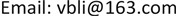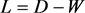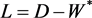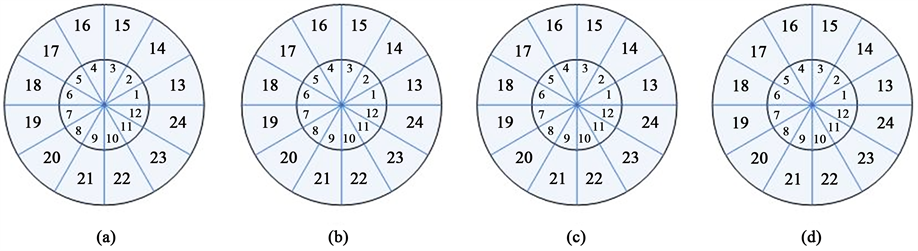﻿ 基于优化图的半监督学习的行人检测 Pedestrian Detection Based on Optimized Semi-Supervised Learning Method

Computer Science and Application
Vol.08 No.07(2018), Article ID:26097,9 pages
10.12677/CSA.2018.87124

Pedestrian Detection Based on Optimized Semi-Supervised Learning Method

Shaohong Yang, Jun Li, Tuozhong Yao

School of Electronic and Information Engineering, Ningbo University of Technology, Ningbo ZhejiangReceived: Jul. 4th, 2018; accepted: Jul. 19th, 2018; published: Jul. 26th, 2018ABSTRACT

Pedestrian detection remains one of the challenging tasks in the area of computer vision. In order to improve the effectiveness of pedestrian detection, this paper proposes a new approach to pedestrian detection. First, the shape context features of each image are represented. Then, we specifically design a novel optimized graph-based semi-supervised learning for pedestrian detection, in which we maximize the average weighed distance between the suggestion box with pedestrians and the suggestion box without pedestrians, and minimize the average weighed distance between the suggestion boxes with pedestrian. Training data insufficiency and lack of generalization of learning method can be resolved. Compared with several other approaches, the experimental results show that this approach performs more effectively and accurately.

Keywords:Pedestrian Detection, Shape Context Features, Machine Learning, Semi-Supervised Learning1. 引言

2. 图的半监督学习理论

${w}_{ij}=\left\{\begin{array}{l}\mathrm{exp}\left(-\frac{{‖{x}_{i}-{x}_{j}‖}^{2}}{\sigma }\right),\text{ }\text{if}\text{ }\text{\hspace{0.17em}}{x}_{i}\ne {x}_{j}\\ 0\text{ }\text{ }\text{ }\text{ }\text{ }\text{ }\text{ }\text{\hspace{0.17em}}\text{\hspace{0.17em}}\text{otherwise}\end{array}$ (1)(2)

${L}^{*}\left(f\right)=\mathrm{min}\left\{\underset{i=1}{\overset{l}{\sum }}L\left(f\left({x}_{i}\right),{y}_{i}\right)\right\}$ (3)

$s\left(f\right)={f}^{\prime }Lf=\underset{i,j=1}{\overset{n}{\sum }}{w}_{ij}{\left(\frac{f\left({x}_{i}\right)}{\sqrt{{d}_{i}}}-\frac{f\left({x}_{j}\right)}{\sqrt{{d}_{j}}}\right)}^{2}$ (4)

$\begin{array}{c}{f}^{*}=\mathrm{arg}\underset{f\left({x}_{i}\right)}{\mathrm{min}}\left\{s+\gamma {L}^{*}\right\}\\ =\mathrm{arg}\underset{f\left({x}_{i}\right)}{\mathrm{min}}\left\{{f}^{\prime }Lf+\gamma \underset{i=1}{\overset{l}{\sum }}L\left(f\left({x}_{i}\right),{y}_{i}\right)\right\}\end{array}$ (5)

3. 基于优化的半监督学习的行人检测算法

3.1. 特征提取和行人候选区域选取

 (6)

3.2. 优化图的半监督学习模型的构建

3.2.1. 构建优化图的半监督行人检测模型

$\mathrm{min}\underset{i=1}{\overset{{l}^{+}}{\sum }}\underset{j=1}{\overset{{l}^{+}}{\sum }}{w}_{i,j}$ (7)

$\mathrm{max}\underset{i=1}{\overset{{l}^{+}}{\sum }}\underset{j={l}^{+}}{\overset{{l}^{-}}{\sum }}{w}_{i,j}$ (8)

$W=\mathrm{arg}\underset{{w}_{i,j}}{\mathrm{min}}\underset{i=1}{\overset{l}{\sum }}\underset{j=1}{\overset{l}{\sum }}{h}_{i,j}{w}_{i,j}$ (9)

${h}_{ij}=\left\{\begin{array}{l}1,\text{ }\text{\hspace{0.17em}}\text{if}\text{\hspace{0.17em}}1\le i,j\le {l}^{+}\\ -1,\text{\hspace{0.17em}}\text{\hspace{0.17em}}\text{if}\text{\hspace{0.17em}}1\le i\le {l}^{+};\text{\hspace{0.17em}}{l}^{+} (10)(11)

3.2.2. 优化图的半监督行人检测模型的分析

$\underset{\omega ,b}{\mathrm{min}}\underset{i=1}{\overset{l}{\sum }}\underset{j=1}{\overset{l}{\sum }}{\left({{w}^{\prime }}_{i,j}-〈\omega \cdot {x}_{i,j}〉-b\right)}^{2}$ (12)

$\begin{array}{l}\underset{w,b}{\mathrm{min}}\text{\hspace{0.17em}}\text{\hspace{0.17em}}\text{\hspace{0.17em}}\frac{1}{2}{‖\omega ‖}^{2}\\ \text{s}\text{.t}\text{.}\text{\hspace{0.17em}}\text{\hspace{0.17em}}\text{\hspace{0.17em}}\text{\hspace{0.17em}}\left(\left(\omega \cdot {x}_{i,j}\right)+b\right)-{{w}^{\prime }}_{i,j}\le \epsilon ,i,j=1,\cdots ,l\\ \text{\hspace{0.17em}}\text{\hspace{0.17em}}\text{\hspace{0.17em}}\text{\hspace{0.17em}}\text{\hspace{0.17em}}\text{\hspace{0.17em}}\text{\hspace{0.17em}}{{w}^{\prime }}_{i,j}-\left(\left(\omega \cdot {x}_{i,j}\right)+b\right)\le \epsilon ,i,j=1,\cdots ,l\end{array}$ (13)

$\begin{array}{l}L\left(\omega ,b,{\alpha }^{\left(*\right)}\right)=\frac{1}{2}{‖\omega ‖}^{2}-\underset{i=1}{\overset{l}{\sum }}\underset{j=1}{\overset{l}{\sum }}{\alpha }_{i,j}\left(\epsilon +{{w}^{\prime }}_{i,j}-\left(\omega \cdot {x}_{i,j}\right)-b\right)\\ \text{ }\text{ }\text{ }\text{ }\text{ }\text{\hspace{0.17em}}\text{ }\text{\hspace{0.17em}}-\underset{i=1}{\overset{l}{\sum }}\underset{j=1}{\overset{l}{\sum }}{\alpha }_{i,j}^{*}\left(\epsilon -{{w}^{\prime }}_{i,j}+\left(\omega \cdot {x}_{i,j}\right)+b\right)\end{array}$ (14)(15)

$\begin{array}{l}\underset{a,{a}^{\left(*\right)}}{\mathrm{min}}\text{\hspace{0.17em}}\text{\hspace{0.17em}}\frac{1}{2}\underset{i=1}{\overset{l}{\sum }}\underset{j=1}{\overset{l}{\sum }}\underset{m=1}{\overset{l}{\sum }}\underset{n=1}{\overset{l}{\sum }}\left({a}_{i,j}^{*}-{a}_{i,j}\right)\left({a}_{m,n}^{*}-{a}_{m,n}\right)\left({x}_{i,j}\cdot {x}_{m,n}\right)-\epsilon \underset{i,j=1}{\overset{l}{\sum }}\left({a}_{i,j}^{*}+{a}_{i,j}\right)\\ \text{s}\text{.t}\text{.}\text{ }\underset{i=1,j}{\overset{l}{\sum }}\left({a}_{i,j}-{a}_{i,j}^{*}\right)=0,\\ \text{ }\text{ }{a}_{i,j}^{\left(*\right)}\ge 0,i,j=1,\cdots ,l\end{array}$ (16)

${w}^{″}\left({x}_{i,j}\right)=\underset{i=1}{\overset{l}{\sum }}\underset{j=1}{\overset{l}{\sum }}\left({\alpha }_{i,j}^{*}-{\alpha }_{i,j}\right)\left(x\cdot {x}_{i,j}\right)+b$ (17)(18)

 (19)

4. 实验结果及分析

 (20)

$\text{precision}=\frac{{N}_{c}}{{N}_{c}+{N}_{f}}$ (21)Figure 1. Log-Polar Transformation. (a) Direction of 0˚ Log-Polar Transformation; (b) Direction of 45˚ Log-Polar Transformation; (c) Direction of 90˚ Log-Polar Transformation; (d) Direction of 135˚ Log-Polar TransformationFigure 2. Log-Polar coordinate histogram

5. 结束语

Pedestrian Detection Based on Optimized Semi-Supervised Learning Method[J]. 计算机科学与应用, 2018, 08(07): 1125-1133. https://doi.org/10.12677/CSA.2018.87124

1. 1. Oren, M., Papageorgiou, C., Sinha, P., et al. (1997) Pedestrian Detection Using Wavelet Template. 17-19 June 1997, San Juan, 193-199.

2. 2. Ojala, T. and Harwood, I. (1996) A Comparative Study of Texture Measures with Classification Based on Feature Dis-tributions. Pattern Recognition, 29, 51-59. https://doi.org/10.1016/0031-3203(95)00067-4

3. 3. Tuzel, O., Porikli, F. and Meer, P. (2008) Pedestrian Detection via Classification on Riemannian Manifolds. IEEE Transactions on Pattern Analysis & Machine Intelli-gence, 30, 1713. https://doi.org/10.1109/TPAMI.2008.75

4. 4. Szarvas, M., Yoshizawa, A., Yamamoto, M., et al. (2005) Pedes-trian Detection with Convolutional Neural Networks. IEEE Proceedings Intelligent Vehicles Symposium, 224-229.

5. 5. Grubb, G., Zelinsky, A., Nilsson, L., et al. (2004) 3D Vision Sensing for Improved Pedestrian Safety. Intelligent Vehicles Symposium, 19-24.

6. 6. Viola, P. and Jones, M.J. (2004) Robust Real-Time Face Detection. International Journal of Computer Vision, 57, 137-154. https://doi.org/10.1023/B:VISI.0000013087.49260.fb

7. 7. Mohan, A., Papageorgiou, C. and Poggio, T. (2001) Exam-ple-Based Object Detection in Images by Components. IEEE Transactions on Pattern Analysis and Machine Intelligence, 23, 349-361. https://doi.org/10.1109/34.917571

8. 8. Felzenszwalb, P.F., Girshick, R.B., Mcallester, D., et al. (2014) Object Detection with Discriminatively Trained Part-Based Models. IEEE Transactions on Pattern Analysis & Machine Intelligence, 47, 6-7.

9. 9. Ali, K., Fleuret, F., Hasler, D., et al. (2009) Joint Pose Estimator and Feature Learning for Object Detection. IEEE International Conference on Computer Vision, 1373-1380.

10. 10. Jung, S.I. and Hong, K.S. (2017) Deep Network Aided by Guiding Network for Pedestrian Detec-tion. Pattern Recognition Letters, 90. https://doi.org/10.1016/j.patrec.2017.02.018

11. 11. Tomè, D., Monti, F., Baroffio, L., et al. (2015) Deep Convolutional Neural Networks for Pedestrian Detection. Signal Processing Image Communication, 47, 482-489.

12. 12. 胡崇海. 基于图的半监督机器学习[D]: [博士学位论文]. 杭州: 浙江大学, 2008.

13. 13. 高隽, 谢昭. 图像理解理论与方法[M]. 北京: 科学出版社, 2009.

14. 14. Belongie, S., Malik, J., et al. (2002) Shape Matching and Object Recognition Using Shape Contexts. IEEE Transactions on Pattern Analysis and Machine Intelligence, 24, 509-522. https://doi.org/10.1109/34.993558

15. 15. Mori, G., Belongi, S., et al. (2005) Efficient Shape Matching Using Shape Contexts. IEEE Transactions on Pattern Analysis and Machine Intel-ligence, 27, 1832-1837. https://doi.org/10.1109/TPAMI.2005.220

16. 16. Uijlings, J.R., Sande, K.E., Gevers, T., et al. (2013) Se-lective Search for Object Recognition. International Journal of Computer Vision, 104, 154-171. https://doi.org/10.1007/s11263-013-0620-5